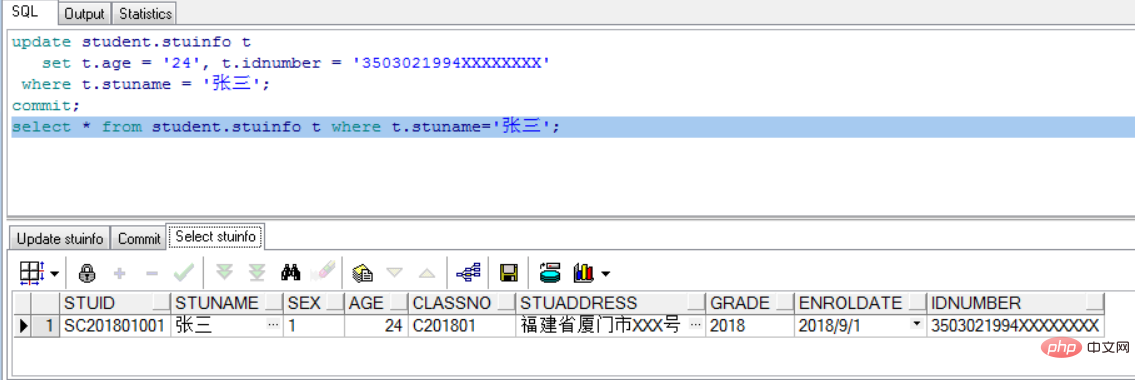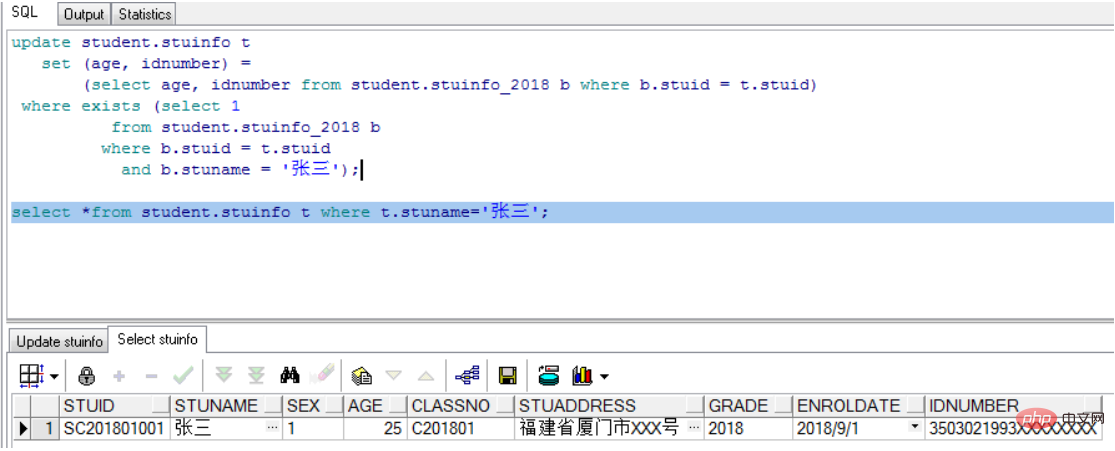oracle如何修改列的值update语句可以用来修改、更新一个或多个表的数据。

update 表名 set 列名1=值1,列名2=值2,列名3=值3..... where 条件

update student.stuinfo t
set t.age = '24', t.idnumber = '3503021994XXXXXXXX'
where t.stuname = '张三';
commit;
select * from student.stuinfo t where t.stuname='张三';update 利用另外一张表关联更新本表数据的命令结构如下：

update 表1 set 列名=（select 列名 from 表2 where 表1.列名=表2.列名）
where exists (select 1 from 表2 where 表1.列名=表2.列名)

update student.stuinfo t
set (age, idnumber) =
(select age, idnumber from student.stuinfo_2018 b where b.stuid = t.stuid)
where exists (select 1
from student.stuinfo_2018 b
where b.stuid = t.stuid
and b.stuname = '张三');

select *from student.stuinfo t where t.stuname='张三';全部评论我要评论

• 取消发布评论发送
• 1/1